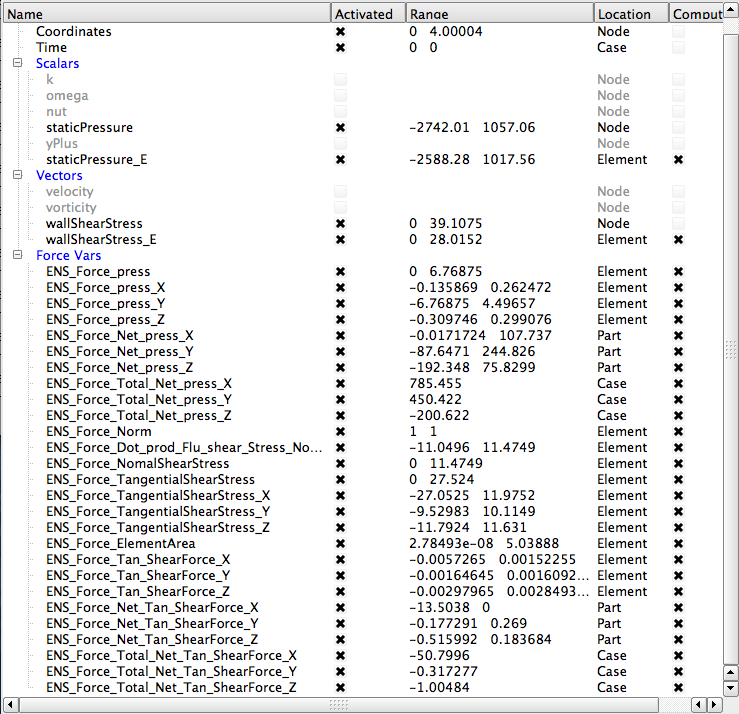﻿ Force Tool

# Force Tool

EnSight now includes a pressure and shear net force calculation tool that replaces the previous pressure force calculation tool.

## Features

• Calculates the net force on a per part basis
• Calculates the net force from pressure and from shear (either  shear force or shear stress)
• Saves the net force component values in part metadata accessible in EnSight python
• Can optionally calculate the pressure coefficients from shear and pressure (using user-supplied reference velocity, density and surface area).
• Can also optionally calculate the lift, drag and side forces (and the corresponding coefficients) from a user-supplied reference velocity and an 'up' vector
• Drag is in the direction of the reference velocity
• Side force is perpendicular to the drag
• Lift is perpendicular to the side force and the drag force with a positive dot product with the 'up' vector
• Saves the forces and coefficients in a user selected, comma-separated value (.csv) format easily imported into a spreadsheet.
• Allows the user to color all parts by a user-selected net force (accessed from the part metadata) with a crude legend for visualization of the net force on each part
• Saves command language for playback
• Has a help button that takes you here.

## Limitations

• Only calculates the net shear force from a shear stress or shear force variable.  Does not use EnSight to calculate these values because we have seen in the past that the solver does a better job of calculating the velocity gradient at the wall.  The solver has the physics, boundary conditions, and internal numerical algorithms designed to best approximate the shear stress or force.  EnSight is best used to analyze, visualize and communicate these results rather than act as  a solver.
• Only works on 2D parts (parts containing tri, quad, or polygon elements). Does not calculate forces on parts with 1D line elements, 3D volume elements nor on point parts
• Does not calculate the reference area, the reference velocity nor the reference density. These must be user-supplied.
• Does not yet calculate the center of pressure.

## Usage

1. Select the part(s) of interest. The parts must only have 2D surface elements.

2. Open the tool box and double click the Force Pressure & Shear tool.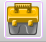You may recognize the icon from the pressure force tool in previous versions of EnSight. This tool completely replaces the functionality of that tool.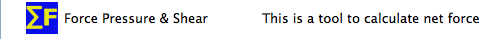3. The tool has three GUIs

a. The First GUI allows four choices for what to calculate in progressive order of complexity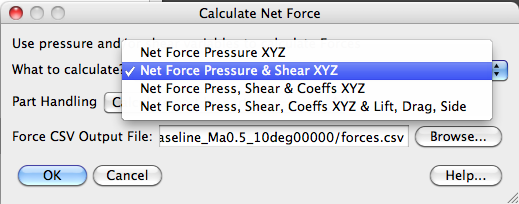• Pressure net force in the XYZ coordinate system
• Pressure and shear net force in the XYZ coordinate system
• Pressure and shear net force as well as the coefficients in the XYZ coordinate system
• Pressure and shear net force in the XYZ coordinate system as well the Lift, Drag, and Side components and all the coefficients

as well as part by part net force calculations or one total net force for all parts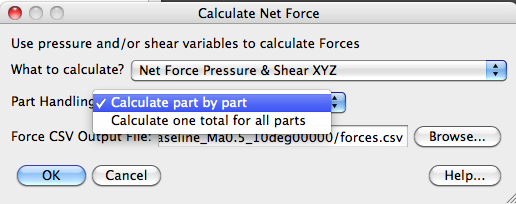and the ability to choose an output .csv file name and path and to browse for an appropriate directory and file name.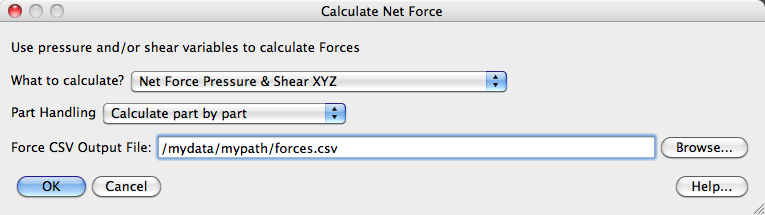b. The Second GUI depends on the choice made in the first GUI

i. Pressure net force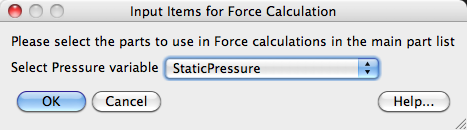ii. Pressure and shear net force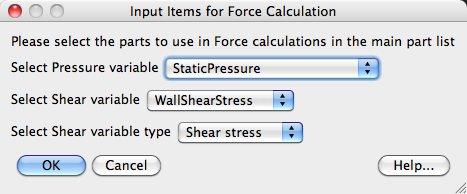iii. Pressure and shear net force as well as the coefficientsiv. Pressure and shear net force as well the Lift, Drag, and Side components and all the components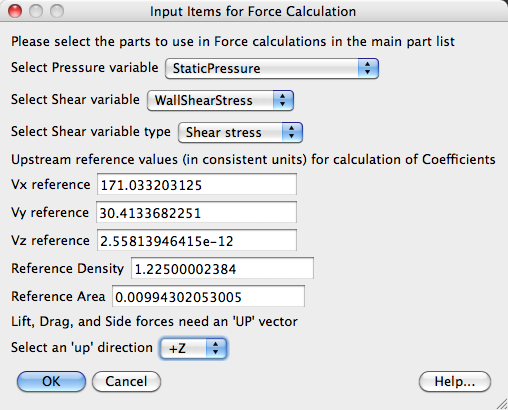Note that the above GUI chooses intelligent defaults using the existing variable names.  For example, as shown in the variable list below, if the variable list contains scalar variables containing "pressure" or "shear" then this tool will auto-select these variables as viable candidates for use in the calculations: it will make these variables the default in the GUI above.  Note also for coefficient calculations that if you have constants with "Area", "Density", "Vx", "Vy", or "Vz" as shown in the variable list below, then the tool will use these variables for the default reference values as shown in the GUI above. This can save time when rerunning the tool, simply as part of the setup, create variables with these names.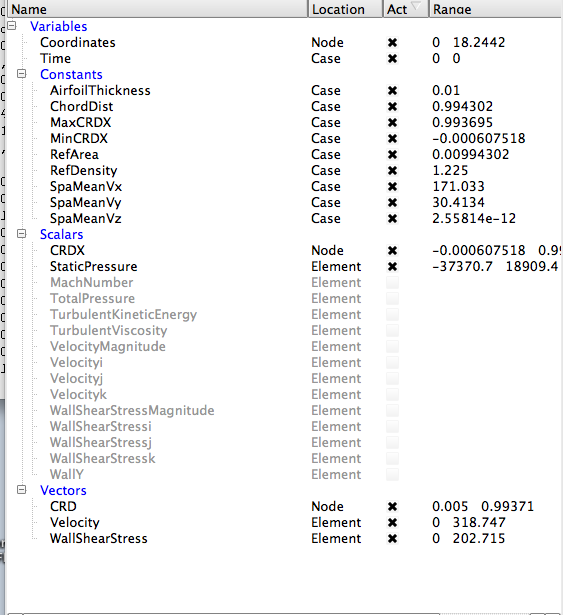c. If you have one part, then the Third GUI informs you that the calculations are complete and where the values are written out.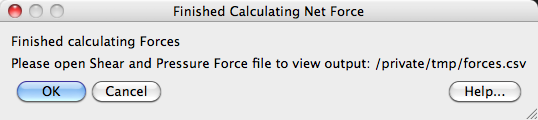d. Notice all of the new variables that are created are put under a Force Vars folder. The variables with "press" in their name are pressure variables. The variables with "ShearForce" in their names are the shear variables.  The remaining variables are intermediate variables. Note that these variables are calculated using the parts selected by the user.

### Pressure variables.

ENS_Force_press is the force vector variable at each element from pressure, and the X, Y, and Z components are the next three.

ENS_Force_Net_press_X, Y, & Z are the per part constant variables that are the net force from pressure at each of the selected parts.  In EnSight 10.2 we now have Per Part constants. This enables us to create a single variable name that has a value for each of the selected parts. You can color the selected parts by the per part variables to see how the Net Force varies by part. You can save your per part variables to a CSV file by right clicking on the variable in the variable list and choosing Export to CSV file.

ENS_Force_Total_Net_press_X, Y, & Z are the Case Constants representing the sum of the pressure force in the x, y, and z directions.

### Shear variables

ENS_Force_Tan_ShearForce_X, Y, & Z are scalar components of the tangential shear force at each element.

ENS_Force_Net_Tan_ShearForce_X, Y, & Z are the per part constants representing the sum of the shear scalar above over all the elements in that part.

ENS_Force_Total_Net_Tan_ShearForce_X, Y, & Z are the Case Constants representing the sum of the shear scalars over all the elements for all selected parts.

And the remaining variables are intermediate variables.